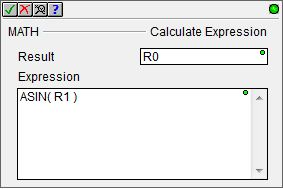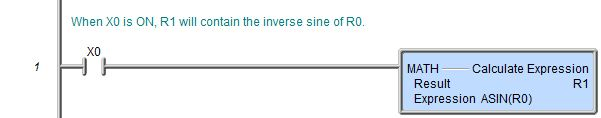Topic: DMD0088

## ASIN - Arcsine in Radians

This instruction can only be used in the Expression parameter of the Calculate Expression (MATH) instruction.

The Arcsine in Radians (ASIN) math function will compute the inverse sine of the function expression.

The expression parameter consists of all the text between the bounding parentheses. Any expression parameter can be a constant value, a bit or numeric memory location, an arithmetic expression, and/or mathematical function or other expressions up to a maximum of 1024 characters. Expressions can be nested, so use of parentheses is recommended to ensure proper evaluation order.

The range of acceptable input values is -1.0 to +1.0. A value outside this range will generate an 'Argument Out of Range' warning - \$OutOfRange (ST132) will be ON.The output value will be in radians, in the range of -1.570796 to +1.570796 (-π/2 to π/2).

Some examples:

ASIN( -1.0 ) = π

ASIN( 0.0 ) = 1.571 (π/2)

ASIN( +1.0 ) = 0.0

If the result needs to be in degrees, use the DEG function on the resulting function, for example, DEG( ASIN( +1.0 ) ) = 90.0 degrees

#### Rung Example: